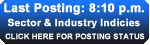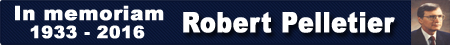# Point and Tick Values

CSI displays 3 different types of price values related to futures markets. Usually these values can be computed from the contract size, units, conversion factor, and minimum tick.

### Full Point Value

This represents the contract value per 1.0 unit of currency.This is the general formula:

FullPointValue = ContractSize x UnitOfMeasurement

For example, CME Live Cattle contract size is 40000 lbs and units are cents per pound.

FullPointValue = ContractSize x UnitOfMeasurement = 40000 lbs x cents per pound = 40000 cents = \$400.00

Alternatively this formula can be used:

FullPointValue = FranctionalPointValue x ConversionFactorDivisor

For example, the CME website contract specifications states that the FractionalPointValue is \$4.00. The conversion factor is +2 which means the ConversionFactorDivisor is 100.

FullPointValue = FranctionalPointValue x ConversionFactorDivisor = \$4.00 x 100 = \$400.00

The conversion factor divisors:
-9 =640
-8 =320
-7 =320
-6 =256
-5 =128
-4 =64
-3 =32
-2 =16
-1 =8
0 =1
1 =10
2 = 100
3 = 1000
4 = 10000
5 = 100000
6 = 1000000
7 = 10000000
8 = 100000000

### Fractional Point Value

This represents the contract value of the pricing increment precision unit.

FranctionalPointValue = FullPointValue / ConversionFactorDivisor

### Tick Value

The tick value is the minimum price fluctuation of one contract.

TickValue = FractionalPointValue x MinimumTick

For example, CME Live Cattle has a minimum tick of 2.5 and the FractionalPointValue is \$4.00.

TickValue = FractionalPointValue x MinimumTick = \$4.00 x 2.5 = \$10.00